Function Repository Resource:

# DropColumn

Drop columns from matrices

Contributed by: Michael Sollami
 ResourceFunction["DropColumn"][mat,n] gives mat with its first n columns dropped. ResourceFunction["DropColumn"][mat,-n] gives mat with its last n columns dropped. ResourceFunction["DropColumn"][mat,{n}] gives mat with its nth column dropped. ResourceFunction["DropColumn"][mat,{m,n}] gives mat with columns m through n dropped. ResourceFunction["DropColumn"][mat,{m,n,s}] gives mat with columns m through n in steps of s dropped. ResourceFunction["DropColumn"][spec] represents an operator form of ResourceFunction["DropColumn"] that can be applied to an expression.

## Details and Options

ResourceFunction["DropColumn"] works with Span as well as the standard sequence specification.
ResourceFunction["DropColumn"] can only be used on tensors of rank 2 or more, e.g. matrices.
ResourceFunction["DropColumn"] does not work with SparseArray objects or with Association.

## Examples

### Basic Examples (1)

Drop the first two columns from a matrix:

 In:=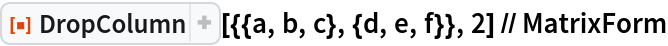Out=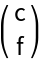### Scope (5)

Drop the third column from a matrix:

 In:=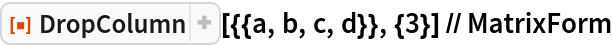Out=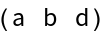Drop the odd columns from a matrix:

 In:=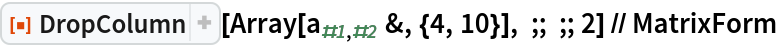Out=Drop the last eight columns from a matrix:

 In:=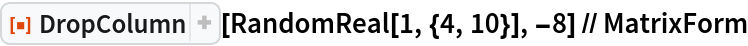Out=Drop the third column from a tensor:

 In:=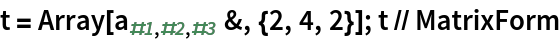Out=In:=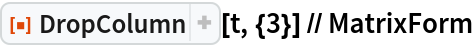Out=Use the operator form of DropColumn:

 In:=Out=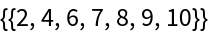Michael Sollami

## Requirements

Wolfram Language 11.3 (March 2018) or above

## Version History

• 1.0.0 – 08 March 2019